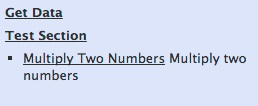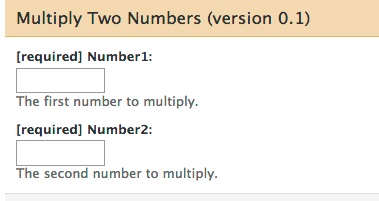# RGalaxy lab ## July 19, 2013 [Galaxy](http://galaxyproject.org/) is an open, web-based platform for data-intensive biomedical research. It provides an easy-to-use web interface and can expose bioinformatics workflows written in any programming language. The `RGalaxy` package allows you to take existing `R` code and expose it in Galaxy, so that anyone with a web browser can run your function without needing any knowledge of `R`. Let's start with a very simple example, based closely on one from the `RGalaxy` vignette. This is a function which multiplies two numbers. It's obviously too trivial to be of use to anyone, but it illustrates some important principles. ```{r multiplyTwoNumbers} library(RGalaxy.lab) multiplyTwoNumbers <- function( number1=GalaxyNumericParam(required=TRUE), number2=GalaxyNumericParam(required=TRUE), product=GalaxyOutput("product", "txt")) { cat(number1 * number2, file=product) } ``` There are a few things to notice about this function: * The data type of each parameter is specified. And instead of just specifying `R`'s `numeric` type, we are using a special class called `GalaxyNumericParam`. This is because Galaxy (unlike `R`) needs to know the type of each parameter, as well as other information * The function's name is descriptive. * The return value of the function is not important. Instead, the function writes information to one or more files. * All the function's inputs and outputs are specified as named arguments in its signature. This is required as Galaxy communicates with tools by sending them files and reading files they generate. * By default, parameters are marked as not required by Galaxy. Adding `required=TRUE` tells Galaxy not to allow empty values. * This function can be run from within R, passing it ordinary `numeric` values: ```{r run_multiplyTwoNumbers} t <- tempfile() multiplyTwoNumbers(2, 7, t) readLines(t, warn=FALSE) ``` ## Documenting the Example We're almost ready to tell Galaxy about our function, but first we need to document it with a manual page. `RGalaxy` will use information in this page to create the Galaxy tool, and the man page will also be useful to anyone who wants to run your function in `R`. This command will open the man page (`multiplyTwoNumbers.Rd`) in `RStudio`'s editor, so you can examine it: ```{r manpage} manpage <- system.file("manpages", "multiplyTwoNumbers.Rd", package="RGalaxy.lab") ``` ```{r open_man_page, eval=FALSE} file.edit(manpage) ``` ```{r open_man_page_real, echo=FALSE} if (identical(.Platform\$GUI, "RStudio")) { file.edit(manpage) } ``` Some things to note about this man page: * The `name`, `alias`, `description`, `title`, `usage`, and `arguments` sections are required. The `details` section is not required but its use is encouraged (`RGalaxy` will notify you if this section is missing). * While it's good to have an `examples` section, this section is only useful to people running your function via `R`. This section is not used by `RGalaxy`. ## Installing Galaxy If you were working on your own machine, you'd need to install Galaxy at this point. You can refer to the `RGalaxy` vignette for information about this procedure. Galaxy is already installed on the virtual machine you are accessing through your browser, so we'll skip the installation step for now. We do however need to tell R where Galaxy is installed: ```{r set_galaxy_home} galaxyHome <- "/extra/ubuntu/galaxy-dist" ``` ```{r set_galaxy_home2, echo=FALSE, results="hide"} hostname <- system2("hostname", stdout=TRUE) if (grepl("^dhcp", hostname)) galaxyHome <- "/Users/dtenenba/dev/galaxy-dist" ``` ## Telling Galaxy about the function Now we point Galaxy to the function we just wrote: ```{r library_RGalaxy_real, echo=FALSE, results="hide"} suppressPackageStartupMessages(library(RGalaxy)) ``` ```{r run_galaxy, tidy=FALSE} library(RGalaxy) galaxy("multiplyTwoNumbers", manpage=manpage, version=0.1, galaxyConfig= GalaxyConfig(galaxyHome, "mytool", "Test Section", "testSectionId") ) ``` ```{r restart_galaxy_function, echo=FALSE} restartGalaxy <- function(galaxyHome) { oldwd <- getwd() on.exit(setwd(oldwd)) setwd(galaxyHome) processes <- system2(c("ps", "-ef"), stdout=TRUE) galaxyProcess <- processes[grepl("paster\\.py", processes)] galaxyProcess <- sub("^ +", "", galaxyProcess) if (length(galaxyProcess) > 1) stop("More than one galaxy process running. Aborting.") if (length(galaxyProcess)==0) { cat("Note: Galaxy is not running.\n") } else { pid <- strsplit(galaxyProcess, " +")[] system2("kill", sprintf("-9 %s", pid)) } system2("nohup", "./run.sh > galaxy.log 2>&1 &") cat("Galaxy will be restarted in a moment.\n") } ``` We've told Galaxy about our function by modifying its configuration files. Each time we do this, we need to restart Galaxy. The `RGalaxy` vignette describes how to do this, but we've defined a function that will do it on this virtual machine. Let's run the function: ```{r call_restartGalaxy} restartGalaxy(galaxyHome) ``` Now we can run Galaxy. The following command will open a new window. If nothing happens, you may need to deactivate the popup blocker in your browser. ```{r getGalaxyUrl, echo=FALSE} getGalaxyUrl <- function(silent=FALSE) { try(hostnamed <- suppressWarnings(system2(c("hostname", "-d"), stdout=TRUE, stderr=NULL)), silent=TRUE) if (exists("hostnamed") && length(hostnamed) && grepl("ec2\\.internal", hostnamed)) { library(httr) public.dns <- content(GET("http://169.254.169.254/latest/meta-data/public-hostname")) url <- paste0("http://", public.dns, ":8080") } else { port <- "8080" hostname <- system2("hostname", stdout=TRUE) if (grepl("^dhcp", hostname)) port <- "8081" url <- sprintf("http://localhost:%s", port) } if (!silent) { cat("You can launch Galaxy with:\n") cat(paste0("browseURL(getGalaxyUrl())\n")) cat("Be sure popup-blockers are disabled.\n") } url } ``` ```{r run_getGalaxyUrl} Sys.sleep(4) browseURL(getGalaxyUrl()) ``` You might need to reload the page in the window that just opened. In the Galaxy window, at upper left, you should see a link called "Test Section". Click on it and you'll see "Multiply Two Numbers":Now click on "Multiply Two Numbers". You can see that all of the information in the middle part of the window comes from either our function, its man page, or the invocation of the `galaxy()` function.Enter two numbers and click "Execute". When the tool is finished running, the "product.txt" link at right will turn green. If you click it, you'll see the result of the multiplication. This is a cumbersome way to multiply two numbers. But it's a useful example. ## Functional Testing We just ran Galaxy and made sure our tool worked. It would be nice to automate this procedure so we can know that for inputs `x` and `y`, our tool will always produce output `d`. With a couple of small additions, we can accomplish this. Our function will have a self-contained test. Also, when submitting tools to the public Galaxy instance, functional tests like this are required. Here is our `addTwoNumbers` function again, this time with a functional test: ```{r multiplyTwoNumbersWithTest, tidy=FALSE} multiplyTwoNumbersWithTest <- function( number1=GalaxyNumericParam(required=TRUE, testValues=5L), number2=GalaxyNumericParam(required=TRUE, testValues=5L), product=GalaxyOutput("sum", "txt")) { cat(number1 * number2, file=product) } ``` The only visible difference is that we've added a `testValues` argument to each input parameter. Another, subtler difference is that we have added a file called `functionalTests/multiplyTwoNumbersWithTest/product`, which contains the expected output of the function. Does the function pass its functional test? ```{r runFunctionalTest} runFunctionalTest(multiplyTwoNumbersWithTest, system.file("functionalTests", package="RGalaxy.lab")) ``` ## A more practical example, using Bioconductor. Suppose you have some Affymetrix probe IDs and you want to look up the PFAM and SYMBOL names for them. It's quite easy to write a function to expose this in Galaxy: ```{r probeLookup, tidy=FALSE} probeLookup <- function( probe_ids=GalaxyCharacterParam( required=TRUE, testValues="1002_f_at 1003_s_at"), outputfile=GalaxyOutput("probeLookup", "csv")) { suppressPackageStartupMessages(library(hgu95av2.db)) ids <- strsplit(probe_ids, " ")[] results <- select(hgu95av2.db, keys=ids, columns=c("SYMBOL","PFAM"), keytype="PROBEID") write.csv(results, file=outputfile) } ``` Behind the scenes, we've also written a man page for the function, and written a test fixture. Let's run it and make sure it works: ```{r test_probeLookup} runFunctionalTest(probeLookup, system.file("functionalTests", package="RGalaxy.lab")) ``` ### Exercise 1 Tell Galaxy about this function and run it in Galaxy. ```{r exercise_1_answer, echo=FALSE, eval=FALSE, tidy=FALSE} galaxy("probeLookup", manpage=system.file("manpages", "probeLookup.Rd", package="RGalaxy.lab"), version=0.1, galaxyConfig= GalaxyConfig(galaxyHome, "mytool", "Test Section", "testSectionId") ) restartGalaxy(galaxyHome) Sys.sleep(4) browseURL(getGalaxyUrl()) ``` ### Exercise 2 Write your own workflow (it could be just a simple function), preferably using Bioconductor, add functional tests and fixtures, and expose it in Galaxy. ```{r exercise_2_answer, echo=FALSE, eval=FALSE} # It's an open-ended question. ``` ## Further Information (Demo of RGalaxy tools) The `RGalaxy` vignette contains much more information about using `RGalaxy`. To open the vignette in command-line R, run this command: ```{r open_RGalaxy_vignette_1, eval=FALSE} browseVignettes("RGalaxy") ``` In RStudio, run this command: ```{r open_RGalaxy_vignette_2, eval=FALSE} help(package="RGalaxy") ``` Then click on "User guides, package vignettes and other documentation", and then click on "RGalaxy::RGalaxy-vignette".